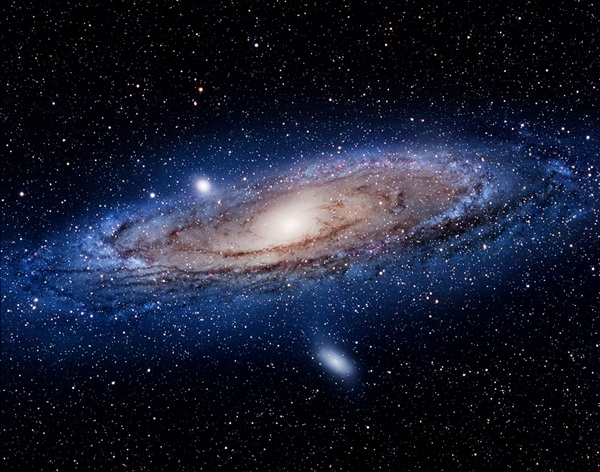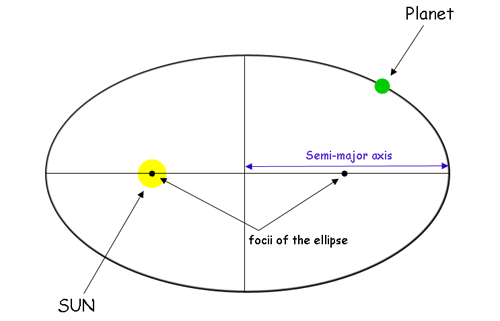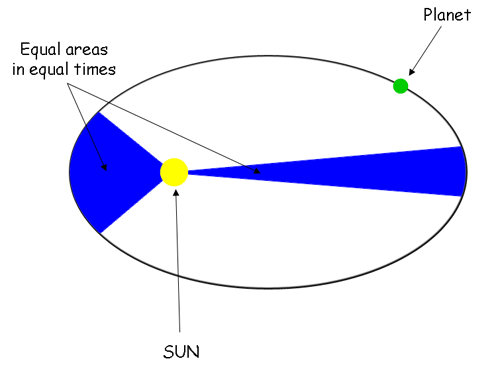Crystal

HS-ESS1-4

# Kepler’s Law Of Planetary Motion Study Guide

Kepler devised a set of three fundamental rules based on the movement of the planets in the solar system, known as the three laws of planetary motion by Kepler.

Table of content

# Introduction

Thousands of particles of debris orbit Earth with many artificial satellites. From the dawn of time, mankind has been fascinated by the moon's orbit around Earth. Planetary systems, asteroids, meteors, and comets all have intriguing orbits around the sun. We can observe practically incalculable numbers of stars, galaxies, and other celestial bodies orbiting one other and interacting through gravity if we look further.Source

## THE PLANETARY MOTIONS AND THEIR NATURE

The definition of planetary motion states the motion of the planets in a sun-centered planetary system. The orbital motions of planets and other celestial bodies in our solar system are straightforward in nature and can be described by a few simple laws.

• The mass of the orbiting object is less than the mass of the object it orbits
• The system is isolated from other bodies.

## THE THREE LAWS OF PLANETARY MOTION BY KEPLER

• Kepler’s first law of planetary motion implies that each planet's orbit around the sun is an ellipse with the sun at one of the foci.
• Perihelion is the planet's closest point to the sun, and aphelion is the planet's furthest distance from the sun.Source

• Kepler’s second law of planetary motion states that each planet moves in such a way that an imaginary line made from the sun to the planet sweeps out the same amount of area in the same amount of time.

• For example, if an imaginary line was formed from the earth to the sun, the region swept out by the line would be the same for every 31-day period.Source

• Kepler’s third law of planetary motion relates a planet's orbital period and radius to other planets.

• The third law, unlike Kepler's first and second principles, compares the motion characteristics of distinct planets.

• The contrast is that for each of the planets, the ratio of the squares of their periods to the cubes of their average distances from the sun is the same.

This Kepler’s law of planetary motion’s equation is

T12 / T22 = R13/ R2.3,

where T is the time for one orbit and R is the orbital radius.

# SUMMARY

• Every planet moves along an ellipse, with the Sun at the ellipse's foci, as per Kepler's first law.
• A planet wipes out identical areas in equal durations, according to Kepler's second law, implying that it has a steady areal velocity.
• The cube of the orbit's semi-major axis is proportional to the square of the period, according to Kepler's third law.

## FAQs

Q. What are the problems with Kepler's law of planetary motion?

The problem with Kepler's law is that it does not explain the concept of Gravitational Force.

Q. What is the significance of Kepler's laws of planetary motion?

The laws of planetary motion established by Kepler signify a watershed moment in the move from geocentrism to heliocentrism. They are the first to establish a quantifiable link between the planets, including Earth.

We hope you enjoyed studying this lesson and learned something cool about Kepler’s Law Of Planetary Motion! Join our Discord community to get any questions you may have answered and to engage with other students just like you! We promise, it makes studying much more fun!😎

## REFERENCE

1. Laws of Planetary Motion: https://flexbooks.ck12.org/cbook/ck-12-physics-flexbook-2.0/section/5.5/primary/lesson/kepler%e2%80%99s-laws-of-planetary-motion-phys/. Accessed 11th April 2022.
2. Kepler’s Law: https://byjus.com/jee/keplers-laws/. Accessed 11th April 2022.